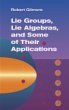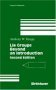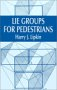# Maths - Algebra Books

IClifford Algebras and Spinors (London Mathematical Society Lecture Note S.)   Pertti Lounesto. This is very complex subject matter, however there is a lot of explanations and it is not all proofs like some mathematical textbooks. The book has a lot of information about the relationship between Clifford Algebras and Hypercomplex Algebras.Clifford Algebra to Geometric Calculus: A Unified Language for Mathematics and Physics (Fundamental Theories of Physics). This book is intended for mathematicians and physicists rather than programmers, it is very theoretical. It covers the algebra and calculus of multivectors of any dimension and is not specific to 3D modelling.Geometric Algebra for Physicists - This is intended for physicists so it soon gets onto relativity, spacetime, electrodynamcs, quantum theory, etc. However the introduction to Geometric Algebra and classical mechanics is useful.Geometric Algebra for Computer Science: An Object-oriented Approach to Geometry. This book stresses the Geometry in Geometric Algebra, although it is still very mathematically orientated. Programmers using this book will need to have a lot of mathematical knowledge. Its good to have a Geometric Algebra book aimed at computer scientists rather than physicists. There is more information about this book here.Contemporary Abstract Algebra written by Joseph Gallian - This investigates algebra (Groups, Rings, Fields) using an abstract (set theory) approach. It is very readable for such an abstract topic but the inclusion of 1 page histories of the great mathematicians and other background information.Introduction to Lie Algebras (Springer Undergraduate Mathematics Series) (Paperback)Lie Groups, Lie Algebras, and Some of Their Applications (Paperback)Lie Groups: Beyond an Introduction (Hardcover)Lie Groups: An Approach through Invariants and Representations (Universitext) (Paperback).Lie Groups for Pedestrians.Engineering Mathematics - This book has been going for a long time and it is now in its 5th edition, so it is tried and tested.

 metadata block see also: Correspondence about this page Book Shop - Further reading. Where I can, I have put links to Amazon for books that are relevant to the subject, click on the appropriate country flag to get more details of the book or to buy it from them.3D Math Primer - Aimed at complete beginners to vector and matrix algebra. Specific to this page here:

This site may have errors. Don't use for critical systems.